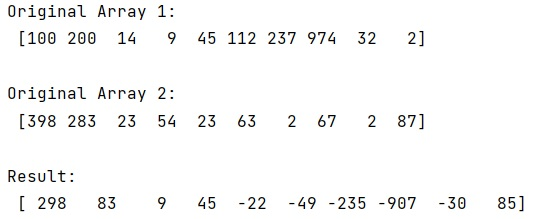# Difference between two NumPy arrays

Learn about the difference between two NumPy arrays in Python.
Submitted by Pranit Sharma, on February 17, 2023

NumPy is an abbreviated form of Numerical Python. It is used for different types of scientific operations in python. Numpy is a vast library in python which is used for almost every kind of scientific or mathematical operation. It is itself an array which is a collection of various methods and functions for processing the arrays.

## Finding the difference between of two NumPy arrays

Suppose that we are given two numpy arrays of integer values and we need to find the difference between these two numpy arrays.

The difference between two numpy arrays is that each value of the second array will get subtracted from its corresponding value in first array.

For this purpose, we will simply subtract the second array from the first array as a whole rather than subtract each value from both arrays.

Let us understand with the help of an example,

## Python code to find the difference between two NumPy arrays

```# Import numpy
import numpy as np

# Creating two numpy arrays
arr1 = np.array([100, 200, 14, 9, 45, 112, 237, 974, 32, 2])
arr2 = np.array([398, 283, 23, 54, 23, 63, 2, 67, 2, 87])

# Display original arrays
print("Original Array 1:\n",arr1,"\n")
print("Original Array 2:\n",arr2,"\n")

# Subtracting arr2 from arr1
res = arr2 - arr1

# Display Result
print("Result:\n",res)
```

Output: